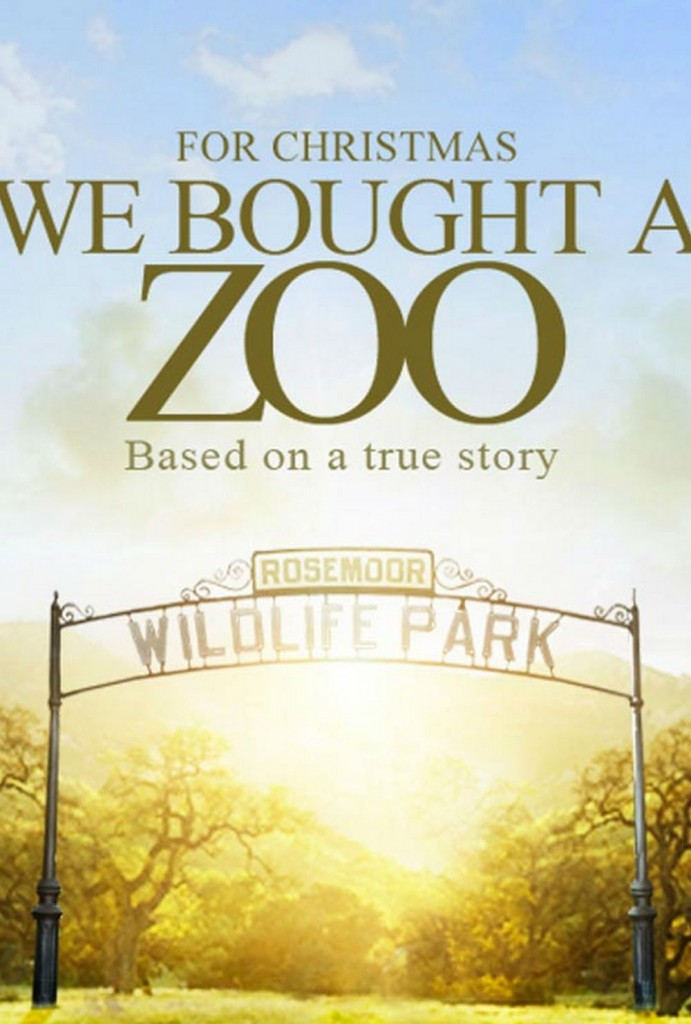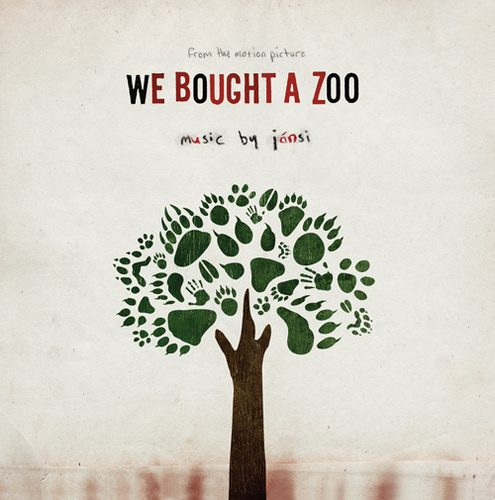### 关注无解音乐网微信# Jónsi 配乐电影《We Bought A Zoo》（我家买了动物园）今天上映TRY:

Jónsi 的原声碟反而先于电影在12月8日就正式发行了，借用Sigur Ros的盛名提前推广电影，不能不说是商家的聪明之举。毕竟《We Bought A Zoo》的导演Cameron Crowe也算和音乐有不解之缘——Crowe最早的一份工作是为美国最著名的音乐杂志Rolling Stone当记者，后来成为经典摇滚电影之一的《Almost Famous》基本是自传性的故事。如你大概可以猜想到的，Jónsi 所做的原声一贯的空灵而宏大，柔情万转弦乐和钢琴，加上他自己独特的嗓音，好像已经让你可以看到荒野上升腾的雾气，已经让你相信故事和爱情即将发生。1. 哥也准备用Wordpress了，以后要常来学习了。

2. 答案妥妥的143547 =。= 思考方式如下~ 2*7=14 7*5=35 14+35-2=47 这题应该不至于2%吧 这概率显得我们国人有多笨样！

3. 答案妥妥的143547 =。= 思考方式如下~ 2*7=14 7*5=35 14+35-2=47 这题应该不至于2%吧 这概率显得我们国人有多笨样！

4. 答案妥妥的143547 =。= 思考方式如下~ 2*7=14 7*5=35 14+35-2=47 这题应该不至于2%吧 这概率显得我们国人有多笨样！

5. 答案妥妥的143547 =。= 思考方式如下~ 2*7=14 7*5=35 14+35-2=47 这题应该不至于2%吧 这概率显得我们国人有多笨样！

6. 答案妥妥的143547 =。= 思考方式如下~ 2*7=14 7*5=35 14+35-2=47 这题应该不至于2%吧 这概率显得我们国人有多笨样！

7. 答案妥妥的143547 =。= 思考方式如下~ 2*7=14 7*5=35 14+35-2=47 这题应该不至于2%吧 这概率显得我们国人有多笨样！

8. 答案妥妥的143547 =。= 思考方式如下~ 2*7=14 7*5=35 14+35-2=47 这题应该不至于2%吧 这概率显得我们国人有多笨样！

9. 答案妥妥的143547 =。= 思考方式如下~ 2*7=14 7*5=35 14+35-2=47 这题应该不至于2%吧 这概率显得我们国人有多笨样！

10. 答案妥妥的143547 =。= 思考方式如下~ 2*7=14 7*5=35 14+35-2=47 这题应该不至于2%吧 这概率显得我们国人有多笨样！

11. 答案妥妥的143547 =。= 思考方式如下~ 2*7=14 7*5=35 14+35-2=47 这题应该不至于2%吧 这概率显得我们国人有多笨样！

12. 答案妥妥的143547 =。= 思考方式如下~ 2*7=14 7*5=35 14+35-2=47 这题应该不至于2%吧 这概率显得我们国人有多笨样！